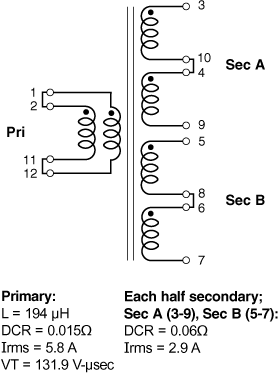## サイトの設定

##### 通貨の設定

RF
EMI
リソース

モデル＆レイアウトツール

ビデオライブラリ
Cx Family Common Mode Chokes
0402CT Low Profile Chip Inductors
XAL7050 High-inductance Shielded Power Inductors
XGL4020 Ultra-low DCR Power Inductors
Quality

リソース

# Application example

for Hexa-Path – Half bridge

<- Back

## Formulas used to calculate electrical characteristics

Connecting windings in series:

• Inductance = Inductance(table) × (number of windings)2
• DCR = DCR(table) × number of windings
• Isat = (Isat(table) × 6) ÷ number of windings connected in series
• Irms = Irms(table)

Connecting windings in parallel:

• Inductance = Inductance(table)
• DCR = 1 ÷ [number of windings × (1 ÷ DCR(table))]
• Isat = (Isat(table) × 6) ÷ number of windings connected in series
• Irms = Irms(table) × number of windings

### Create a 100 Watt, 1:2, half bridge forward converter transformer with center tapped secondary

Choose HPH6-2400L
Vin = 36 – 57 Vdc; Vout = 24 V, 4.2 A

Part number Inductance (µH) Percent Tolerance DCR max (Ω) Volt-time product (V-µs) Irms (A)
HPH6-2400L_ 194 25 0.03 131.9 2.9

Connecting primary windings in parallel:
When primary windings (Wpri) are connected in parallel, DCR decreases, Irms increases, and inductance and volt-time product remain the same.

Example: For HPH6-2400L, connect two primary windings in parallel:
• Inductance = Inductance(table) = 194 μH
• DCR = 1 ÷ [Wpri × (1 ÷ DCR(table))] = 1 ÷ [(2 × (1 ÷ 0.030]) = 0.015 Ohms
• VT = VT(table) = 131.9 V-μsec
• Irms = Irms(table) × Wpri = 2.90 × 2 = 5.8 AConnecting secondary windings in parallel:
When secondary windings (Wsec) are connected in series, Irms remains the same, but DCR increases.

Example: For HP6-2400L, connect four secondary windings in series, creating a center tap at pins 9 and 5. For each half of the secondary:
• DCR = DCR(table) × Wsec = 0.030 × 2 = 0.060 Ohms
• Irms = Irms(table) = 2.90 A